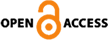### Past Issues

HOMEPast Issues Past Issues

Asian Review of Financial Research Vol.28 No.2 pp.163-195
Predicting Bond Excess Returns in the Korean Market
Key Words : Bond Excess Returns,Predictive Regression,Forward Rate Factor,Inflation Cycle Factor,Output Gap

### Abstract

The analysis of long-term bond excess returns is an important issue in portfolio management and risk dynamics. Under the classical expectation hypothesis, which assumes that long-term yield represents future short-term rate expectations, the excess return on a long-term maturity bond is constant over time. However, it is well known that this assumption does not hold empirically. In the U.S. and other international markets, the forward rate factor suggested by Cochrane and Piazzesi (2005) strongly predicts bond excess returns. Thus, the bond excess return is time-varying and the current yield curve contains information on bond risk premia. Given that the bond price reflects the market participants' expectations regarding future economic states, it should be closely related to macroeconomic variables, such as inflation and real growth. There is a growing area of research called “macro-finance” that tries to explain the term structure of interest rates and bond risk premia with macroeconomic variables. In this paper, we empirically investigate the predictability of bond excess returns in the Korean market. We use information from the current yield curve, inflation shock, and real production shock. Specifically, we use the forward rate factor from Cochrane and Piazzesi (2005), the inflation cycle factor from Cieslak and Povala (2014) and the output gap factor from Cooper and Priestley (2009) as candidate variables to predict bond excess returns. First, we construct the three candidate factors: forward rate, inflation cycle and output gap. The forward rate factor is defined as the fitted value from the regression of the mean excess return on the forward rates. Under the Fisher hypothesis, a long-term yield can be decomposed into an expectation hypothesis term, a long-term inflation expectation term, and a cycle term. The inflation cycle factor summarizes information in the residuals from the regressions of the yields on the long-term inflation expectation. The output gap factor is generated by removing the time trend from the industrial production index. The empirical result shows that the forward rate factor strongly predicts the excess returns of all maturities, with an adjusted   higher than 50%. Consistent with the other markets' results, the information in the yield curve is important in predicting bond excess returns. Compared to the forward rate factor, the inflation cycle and output gap factors, which are individual macroeconomic variables, have weak or no predictive ability. Although the cycle factor has comparable forecasting ability in relation to the forward rate factor, it does not have any additional predictive power when the forward rate factor is taken into account. Even worse, the output gap factor does not have any explanatory power alone. Overall, the results indicate that the macroeconomic variables in our analysis do not have information above the yield curve. We also conduct further analyses of the forward rate factor, which exhibits strong predictive power. The explanatory power of the forward rate factor is beyond the conventional yield curve factors (level, slope and curvature). It does not come from the multicollinearity of forward rates. In addition, the forward rate factor survives after controlling for the effect of international variables; specifically, the U.S. forward rate factor and the currency swap rate. As with the results from the international market data in Sekkel (2011), the explanatory power of the forward rate factor decreased after the financial crisis. Finally, the forward rate factor effectively explains the longer maturity bonds with maturities longer than five years. Focusing on the failure of the inflation cycle factor, which theoretically contains refined information about bond risk premia, we find that the long-term inflation expectation does not have explanatory power in addition to bond yields. Cieslak and Povala (2014) argue that the forward rate factor is a restricted version of the cycle factor, and they remove the long-term inflation term from the bond yields. In contrast, the inflation cycle factor becomes a noisier measure of bond risk premia in the Korean market and the predictive power of the forward rate factor is very strong in our sample period in the global market. The empirical results in this study imply the possibility that bond prices in the Korean market can be expressed as an affine model of yield curve factors, as in Cochrane and Piazzesi (2005). Although the macroeconomic factors do not seem to contain information about bond risk premia above the yield curve, we do not strongly reject the macro-finance models from our empirical results, as the macroeconomic factors in this paper are from a small subset of macroeconomic variables. We remain open to the possibility of more explanatory macroeconomic variables of bond risk premia.
LIST
Export citation